# NCERT solutions for class 10 Science: Chapter 12 electricty Intext Questions

In this page we have NCERT solutions for class 10 Science: Chapter 12 electricty Intext Questions . Hope you like them and do not forget to like , social share and comment at the end of the page.
Question 1.
What does an electric circuit mean?
Electric circuit is a continuous and closed path for electric current. It consists of of cell (or a battery), a plug key, electrical component(s), connecting wires etc. .
Question 2. Define the unit of current.
Answer. Unit of current is Ampere. If one Coulomb of charge flows through any section of a conductor in one second then current through it is said to be 1 Ampere.
1 A = 1Cs-1

Question 3.
Calculate the number of electrons constituting one coulomb of charge.
Solution.
We know that charge q on a body is always denoted by
q = ne
where n is any integer positive or negative
If q=1C and e= 1.6 ×10-19C (negative charge on electron)
Then,
$n= \frac {q}{e} = \frac {1}{1.6 \times 10^{-19}}$
Or,
$n= \frac {100}{16} \times 10^{18}= 6.2 \times 10^{18}$
So,
$n=6.2 \times 10^{18} \; electrons$
This way we can calculate the number of electrons on charged body if we know the total charge on the charged body.

Question 4.
Name a device that helps to maintain a potential difference across a conductor.
Potential difference is the difference in electric pressure which results in the flow of electrons. Potential difference across the conductor is maintained by the sources of electricity such as cell, battery, power supply etc.

Question 5.
What is meant by saying that the potential difference between two points is 1 V?
If 1 Joule of work is required to move charge of 1C from one point to another , then it is said that the potential difference between the two points is 1V.

Question 6.
How much energy is given to each coulomb of charge passing through a 6 V battery?
Here we have to remember that “amount of work required to move each coulomb of charge is equal to energy given to it”.
The expression to be used in solving this question is
$\text{Potential Difference} = \frac {\text{work done}}{Charge}$
or
$\text{work done}=\text{Potential Difference} \times charge$
Here according to question, Charge = 1C and Potential difference=6V
So, Work done = 6×1= 6J
Therefore, 6J of energy is given to each Coulomb of charge passing through a battery of 6 V.

Question 7.

On what factors does the resistance of a conductor depend?
Answer. The resistance of a conductor depends on following factors.
• Material of the conductor
• Temperature of the conductor
• Length of the conductor
• Area of cross-section of the conductor

Question 8.
Will current flow more easily through a thick wire or a thin wire of the same material, when connected to the same source? Why?
Resistance of a wire is given by the relation
$R= \rho \frac {l}{A}$
Where $\rho$ = resistivity of the material of the wire
l= length of the wire
A= Area of the cross section of the wire
From above equation we can see that resistance is inversely proportional to the area of cross-section of the wire. Thick wire means more area of cross-section and lower the resistance of wire. Similarly thin wire means less area of cross-section and wire would have higher resistance.
Therefore, current can flow more easily through thick wire than a thin wire.

Question 9.
Let the resistance of an electrical component remains constant while the potential difference across the two ends of the component decreases to half of its former value. What change will occur in the current through it?
From Ohm’s Law we have
$V=IR$
$I= \frac {V}{R}$
where
R= Resistance of the electric component
V= Potential difference
I=Current
Now according to question the resistance of an electrical component remains constant while the potential difference across the two ends of the component decreases to half of its former value. So, we now have
$R_{new}=R$
$V_{new} = \frac {V}{2}$
$I_{new}$ = new amount of current
Therefore from Ohm’s law we can obtain the $I_{new}$ as
$I_{new}= \frac {V_{new}}{R} =\frac {V/2}{R} = \frac {1}{2} \frac {V}{R} = \frac {I}{2}$
Therefore the amount of current flowing through the electric component is reduced to half.
Note:- I have solved this question for the sake of clarity. You can also solve it analytically using proportionality of current and voltage according to Ohm’s Law.

Question 10.
Why are coils of electric toasters and electric irons made of an alloy rather than a pure metal?
Coils of electric toasters and electric iron are made of an alloy rather then a pure metal because,
(a) resistivity of an alloy is generally higher then that of constituent metals.
(b) alloys have high melting point.
(c) alloys do not oxidize.

Question 11.
Use the data in Table given below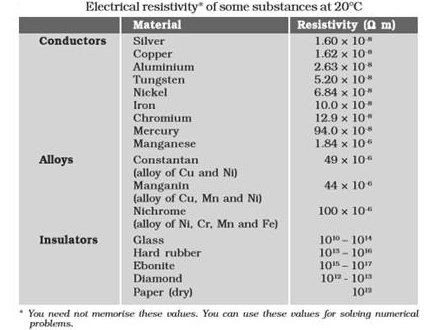(a) Which among iron and mercury is a better conductor?
(b) Which material is the best conductor?
(a) Resistivity of iron = 10.0 x 10-8
Resistivity of mercury = 94.0 x 10-8
Comparing the resistivity of both iron and mercury we conclude that ,  resistivity of mercury is more than that of iron. This implies that iron is a better conductor than mercury.
(b) It can be observed from above Table that the resistivity of silver is the lowest among the listed materials. Hence, it is the best conductor.

Question 12.
Draw a schematic diagram of a circuit consisting of a battery of three cells of 2 V each, a 5Ω  resistor, an 8Ω resistor, and a 12Ω  resistor, and a plug key, all connected in series.
Three cells of potential 2 V, each one of these cells are connected in series therefore the potential difference of the combined battery will be
2 V + 2 V + 2 V = 6V.
The following circuit diagram shows three resistors of resistances 5Ω , 8Ω  and 12Ω respectively connected in series and a battery of potential 6 V and a plug key which is closed means the current is flowing in the circuit.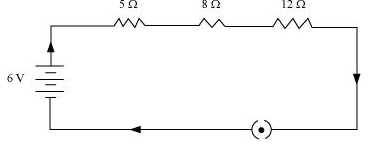Question 13
Redraw the circuit of question 1, putting in an ammeter to measure the current through the resistors and a voltmeter to measure potential difference across the 12Ω resistor. What would be the readings in the ammeter and the voltmeter?
An ammeter should be connected in the circuit in series with the resistors. To measure the potential difference across the resistor it should be connected in parallel, as shown in the following figure.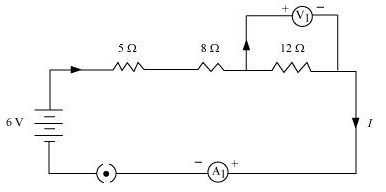The resistances are connected in series.Ohm's law can be used to obtain the readings of ammeter and voltmeter. According to Ohm's law,
V = IR,
Where, Potential difference, V = 6 V
Current flowing through the circuit/resistors = I
Resistance of the circuit, R = 5Ω + 8Ω + 12Ω = 25Ω
I = V/R = 6/25 = 0.24 A
Potential difference across 12Ω  resistor = V1
Current flowing through the 12Ω  resistor, I = 0.24A
Therefore, using Ohm's law, we obtain
V1 = IR = 0.24 x 12 = 2.88 V
Therefore, the reading of the ammeter will be 0.24 A.
The reading of the voltmeter will be 2.88 V.

Question 14
Judge the equivalent resistance when the following are connected in parallel - (a) 1Ω and 106Ω, (b) 1Ω and 103Ω and 106Ω.
(a) when 1Ω and 106Ω are connected in parallel:
We know that for parallel combination of resistances equivalent resistance is given by the relation
$\frac {1}{R} =\frac {1}{R_1} + \frac {1}{R_2}$
If R is the equivalent resistance of the parallel combination then
$\frac {1}{R} = 1 + \frac {1}{10^6}$
Or,
$R= \frac {10^6}{10^6 + 1} \sim \frac {10^6}{10^6}=1$

Therefore, equivalent resistance is nearly equal to 1Ω.
(b) When 1Ω, 103Ω and 106Ω are connected in parallel:
$\frac {1}{R} = \frac {1}{R_1} + \frac {1}{R_2} + \frac {1}{R_3}$
Let R be the equivalent resistance
$\frac {1}{R} = \frac {1}{1} + \frac {1}{10^3} + \frac {1}{10^6} = \frac {10^6 + 10^3 + 1}{10^6}$
0r,
$R=.99 \Omega$
Which is the equivalent resistance of given resistances.
Hence, in both given cases , the equivalent resistance is less than 1Ω

Question 15.
An electric lamp of 100Ω , a toaster of resistance 50Ω , and a water filter of resistance 500 Ω are connected in parallel to a 220 V source. What
is the resistance of an electric iron connected to the same source that takes as much current as all three appliances, and what is the current through it?
Resistance of electric lamp, R1 = 100Ω
Resistance of toaster, R2 = 50Ω
Resistance of water filter, R3 = 500Ω
Potential difference of the source, V = 220V
It is given in the question that these are connected in parallel combination. Equivalent resistance of resistances in parallel combination is
$\frac {1}{R} = \frac {1}{R_1} + \frac {1}{R_2} + \frac {1}{R_3}$
Let R be the equivalent resistance of the circuit.
$\frac {1}{R} = \frac {1}{R_1} + \frac {1}{R_2} + \frac {1}{R_3}= \frac {1}{100} + \frac {1}{50} + \frac {1}{500} = \frac {16}{500}$
or
$R= \frac {500}{16} \Omega$
According to Ohm’s Law , V=IR
Or,
$I = \frac {V}{R}$
Where I is the current flowing through the circuit. So, we have
$I = \frac {220}{500/16} = 7.04 A$
Therefore the current drawn by an electric iron connected to the same source of potential 220 V = 7.04 A
Let R’ be the resistance of the electric iron. According to Ohm’s law,
$V= IR^{'}$
$R^{'} = \frac {220}{7.04}= 31.25 \Omega$
Therefore the resistance of the electric iron is 31.25Ω and the current flowing through it is 7.04 A.

Question 16.
What are the advantages of connecting electrical devices in parallel with the battery instead of connecting
them in series?
Advantages of connecting electrical devices in parallel are:-
1. There is no division of voltage among the appliances when connected in parallel. The potential difference across each appliance is equal to the supplied voltage.
2. The total effective resistance of the circuit can be reduced by connecting electrical appliances in parallel.
3. If one appliance fail to work, other will continue to work properly if they are connected in parallel combination.

Question 17.
How can three resistors of resistances 2Ω , 3Ω  and 6Ω be connected to give a total resistance of (a) 4Ω , (b) 1Ω?
There are three resistors of resistances 2Ω , 3Ω , and 6Ω respectively.
(a) In order to get 4Ω , resistance 2Ω resistance should be connected in series with the parallel combination of 3Ω and 6Ω resistances as shown below in the figure.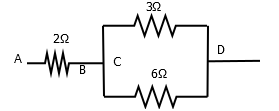$\frac {1}{R_{CD}} = \frac {1}{3} + \frac {1}{6} = \frac {1}{2}$
$R_{CD} =2 \Omega$
and $R_{AB} = 2 \Omega$
This gives
$R_{AD} = R_{AB} + R_{CD} = 2 + 2 =4 \Omega$
(b) In order to get 1Ω all three resistors should be connected in parallel combination as shown in this figure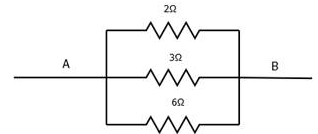Equivalent resistance would be
$\frac {1}{R}= \frac {1}{2} + \frac {1}{3} + \frac {1}{3} = \frac {3+2+1}{6} =1$ $R= 1 \Omega$

Question 18.
What is (a) the highest, (b) the lowest total resistance that can be secured by combinations of four coils of resistance 4Ω , 8Ω , 12Ω , 24Ω ?
(a) The highest resistance is secured by combining all four coil of resistances in series.
$R_S = 4 +8 + 12 +24 =48 \Omega$
(b) If these coils are connected in parallel, then the equivalent resistance will be the lowest, given by
$\frac {1}{R_P} = \frac {1}{4} + \frac {1}{8}+ \frac {1}{12} + \frac {1}{24}=\frac {12}{24}$
$R_p= 2 \Omega$

Question 19.
Why does the cord of an electric heater not glow while the heating element does?
The heating element of the heater is made up of alloy which has very high resistance so when current flows through the heating element, it becomes too hot and glows red. But the resistance of cord which is usually of copper or aluminum is very law so it does not glow.
Also heat produced ‘H’ is given by the relation
$H=I^2 Rt$
Thus, for same amount of current
$H \; \alpha R$
From this we can conclude that more heat is produced by the heating element as it has more resistance, this is the reason it glows.

Question 20.
Compute the heat generated while transferring 96000 coulomb of charge in one hour through a potential
difference of 50 V.
Given Charge, Q = 96000C,
Time, t= 1hr
Potential difference, V= 50 Volts
Now we know that
$H=I^2 Rt= VIt=VQ$
$H= 50 \times 96000=48 \times 10^5 J$

Question 21.
An electric iron of resistance 20Ω takes a current of 5 A. Calculate the heat developed in 30 s.
It is given that
R=20Ω ; I= 5A and t=30s
$H=I^Rt= (5)^2 \times 30 \times 30 =15000J=1.5 \times 10^4 J$

Question 22.
What determines the rate at which energy is delivered by a current?
The rate of consumption of electric energy in an electric appliance is called electric power. Hence, the rate at which energy is delivered by a current is the power of the appliance.

Question 23.
An electric motor takes 5 A from a 220 V line. Determine the power of the motor and the energy consumed in 2 h.
Power (P) is given by the expression,P = VI
Where,
Voltage,V = 220 V
Current, I = 5 A
P= 220 x 5 = 1100 W
Energy consumed by the motor = Pt
Where,
Time, t = 2 h
Energy consumed = VIt = Pt = 1100 x 2 = 2200 Wh